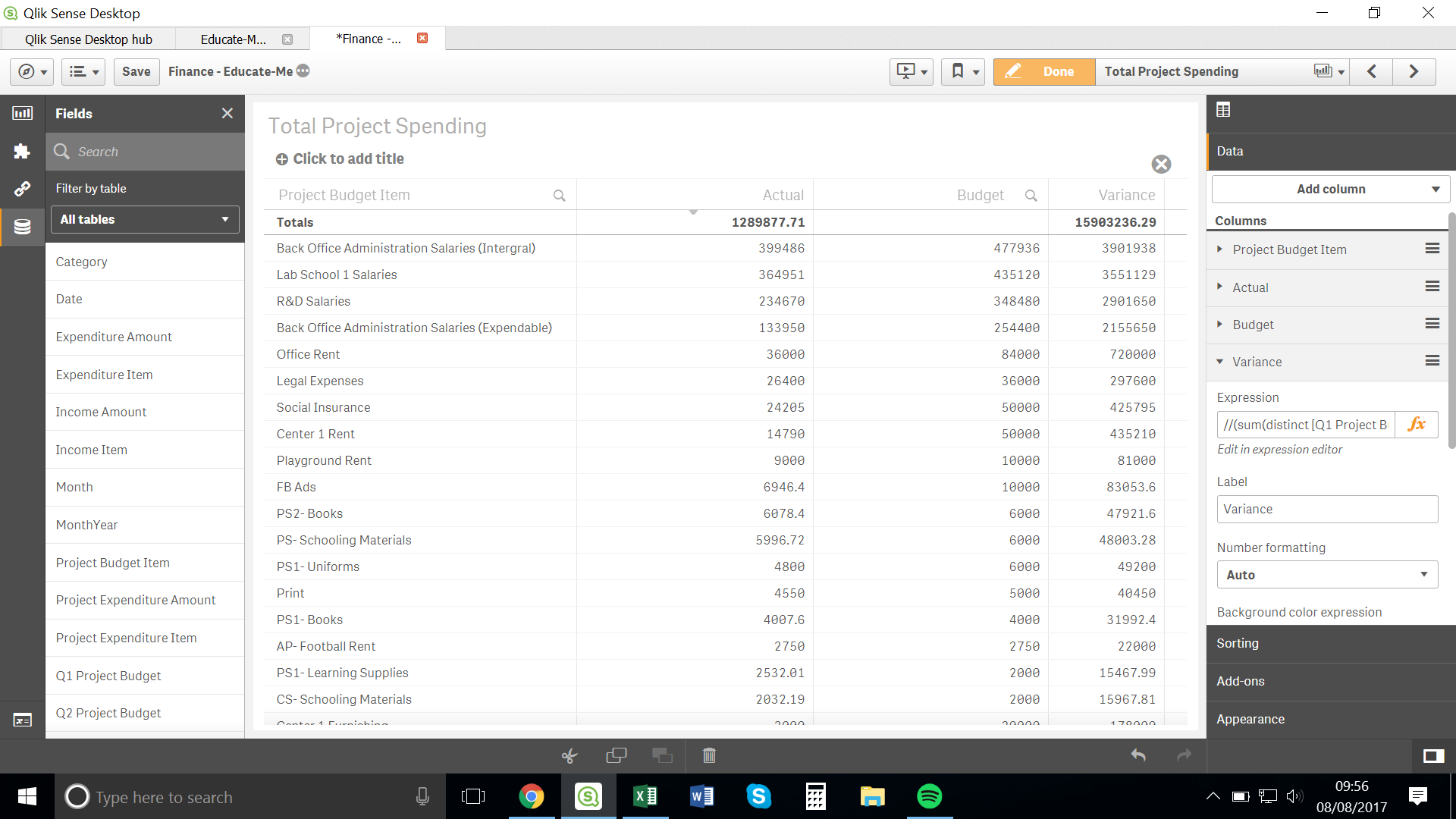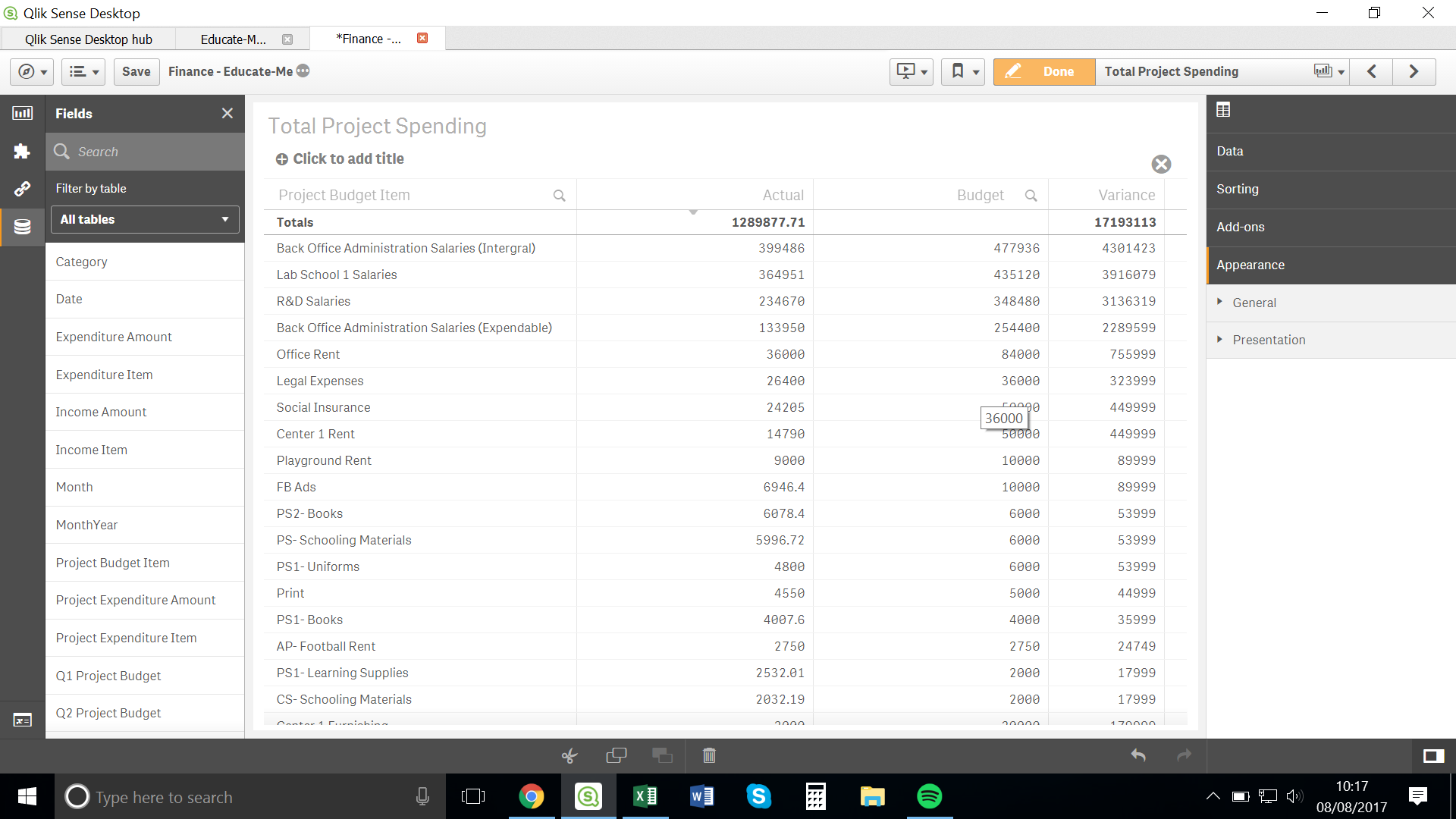# New to Qlik Sense

Discussion board where members can get started with Qlik Sense.

HighlightedNot applicable

## Variance Calculation

Hello All,

I fear that this is a rather foolish question, nevertheless...

I have the following table:I would like to calculate and so I am using the following calculation:

(sum([Q1 Project Budget])+sum([Q2 Project Budget])+sum([Q3 Project Budget])+sum([Q4 Project Budget])) - (sum([Project Expenditure Amount]))

However, it is not yielding the correct answer. Please can someone tell me why this is the case?

Thank you all in advance,

Alison

Tags (4)
1 Solution

Accepted SolutionsNot applicable

## Re: Variance Calculation

I removed sum before the Project Budget and it works.

So the expression is this:

(([Q1 Project Budget]) + ([Q2 Project Budget]) + ([Q3 Project Budget]) + ([Q4 Project Budget]) - sum([Project Expenditure Amount]) )

/ sum([Project Expenditure Amount])

It now works!

Why was sum causing issues?

10 Replies
Contributor III

## Re: Variance Calculation

Hi,

Check this variance formula:-

sum(Actual)-sum(Budget)/Sum(Budget)

Or post sample data or Expr

Regards,

Valued Contributor III

## Re: Variance Calculation

Could you please share the used expression for variance field? Also state the expected result for one of the Project Budget Item.

Generally, I followed below expression (It is purely considering business requirement at my end):

= ((Sum(Budget) - Sum(Actual))/Sum(Actual)) * 100

Regards!

Rahul PawarPartner

## Re: Variance Calculation

better like this:

( sum(Actual)-sum(Budget) ) / Sum(Budget)

and format it as percentage.Not applicable

## Re: Variance Calculation

Hello Lakshmipathi and Rahul,

Sorry, I didn't post the expression. I have done so now.

For Back Office Administration Salaries (Integral) I am expecting 78,450 to be the result.

Using the calculation you have both suggested, which in my app looks like this:

(sum([Q1 Project Budget]) + sum([Q2 Project Budget]) + sum([Q3 Project Budget]) + sum([Q4 Project Budget]) - sum([Project Expenditure Amount])

/ sum([Project Expenditure Amount]))

I get the following result, which is incorrect.Is there anything wrong with the calculation? Or do you imagine that the error lies elsewhere?

Contributor III

## Re: Variance Calculation

Hi,

Try this,

(sum([Q1 Project Budget]) + sum([Q2 Project Budget]) + sum([Q3 Project Budget]) + sum([Q4 Project Budget]) - sum([Project Expenditure Amount]))

/

sum([Project Expenditure Amount])

Regards,Partner

## Re: Variance Calculation

i think you have a problem with brackets:

try this one:

( sum([Q1 Project Budget]) + sum([Q2 Project Budget]) + sum([Q3 Project Budget]) + sum([Q4 Project Budget]) - sum([Project Expenditure Amount]) )

/ sum([Project Expenditure Amount])Not applicable

## Re: Variance Calculation

Hey Andrea,

Thank you for response.

I have tried that calculation and I am still getting the wrong result:

For example:

Actual                    Budget                    Variance

Back Office Administration Salaries (Integral)         399486                   477936                    9.76739...

The Actual and Budget are both correct.

But the variance is not. By your calculation it should be 0.196377...

I don't know what's going on. I feel that the data mustn't be loaded incorrectly or else the acutal and budget columns wouldn't be correct. But I also think there's no issue with the expression you've offered. What's going on?Not applicable

## Re: Variance Calculation

I removed sum before the Project Budget and it works.

So the expression is this:

(([Q1 Project Budget]) + ([Q2 Project Budget]) + ([Q3 Project Budget]) + ([Q4 Project Budget]) - sum([Project Expenditure Amount]) )

/ sum([Project Expenditure Amount])

It now works!

Why was sum causing issues?Partner

## Re: Variance Calculation

is not so easy to help you deeper without a sample app.